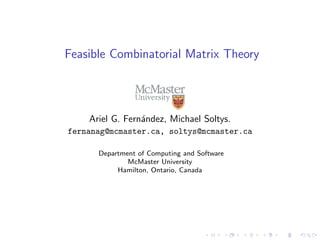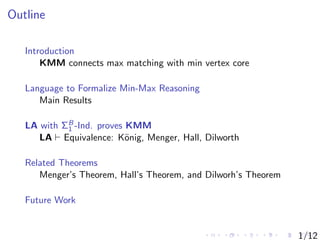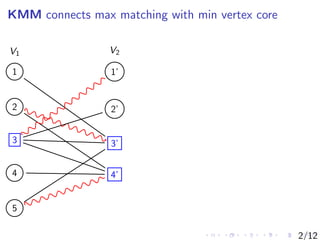Successfully reported this slideshow.

# Feasible Combinatorial Matrix Theory - LICS2013 presentation×

1 of 18
1 of 18

# Feasible Combinatorial Matrix Theory - LICS2013 presentation

This presentation was given by Ariel Fernandez as a short presentation at LICS'13, June 28, 2013, at Tulane University in New Orleans.

This presentation was given by Ariel Fernandez as a short presentation at LICS'13, June 28, 2013, at Tulane University in New Orleans.

## More Related Content

### Feasible Combinatorial Matrix Theory - LICS2013 presentation

1. 1. Feasible Combinatorial Matrix Theory Ariel G. Fern´andez, Michael Soltys. fernanag@mcmaster.ca, soltys@mcmaster.ca Department of Computing and Software McMaster University Hamilton, Ontario, Canada
2. 2. Outline Introduction KMM connects max matching with min vertex core Language to Formalize Min-Max Reasoning Main Results LA with ΣB 1 -Ind. proves KMM LA Equivalence: K¨onig, Menger, Hall, Dilworth Related Theorems Menger’s Theorem, Hall’s Theorem, and Dilworh’s Theorem Future Work 1/12
3. 3. KMM connects max matching with min vertex core 1 2 3 4 5 1’ 2’ 3’ 4’ V1 V2 2/12
4. 4. KMM connects max matching with min vertex core 1 2 3 4 5 1’ 2’ 3’ 4’ V1 V2 M is a Matching denoted by snaked lines. C is a Vertex cover denoted by square nodes. Here M is a Maximum Matching and V is a Minimum Vertex Cover. So by K¨onig’s Mini-Max Theorem, |M| = |C|. 2/12
5. 5. Language to Formalize Min-Max Reasoning LA is (Developed by Cook and Soltys.) Part of Cook’s program of Reverse Mathematics. Three sorts: indices ring elements matrices LA formalize linear algebra (Matrix Algebra). LA over Z (though all matrices are 0-1 matrices.) Since we want to count the number of 1s in A by ΣA. 3/12
6. 6. LA with ΣB 1 -Induction LA (i.e., LA with ΣB 0 -Induction), proves all the ring properties of matrices (eg.,(AB)C = A(BC)), and LA over Z translates into TC0 -Frege ([Cook-Soltys’04]). Bounded Matrix Quantiﬁers: We let (∃A ≤ n)α stands for (∃A)[|A| ≤ n ∧ α], and (∀A ≤ n)α stands for (∀A)[|A| ≤ n → α]. LA with ΣB 1 -Induction correspond to polytime reasoning and proves standard properties of the determinant, and translate into extended Frege. 4/12
7. 7. Main Results Theorem 1: LA with ΣB 1 -Induction KMM. Theorem 2: LA proves the equivalence of fundamental theorems: K¨onig Mini-Max Menger’s Connectivity Hall’s System of Distinct Representatives Dilworth’s Decomposition 5/12
8. 8. LA with ΣB 1 -Ind. proves KMM Diagonal Property ∗ ∗ 0 ... 00 0 0 . . .1 Either Aii = 1 or (∀j ≥ i)[Aij = 0 ∧ Aji = 0]. Claim Given any matrix A, ∃LA proves that there exist permutation matrices P, Q such that PAQ has the diagonal property. 6/12
9. 9. LA Equivalence: K¨onig, Menger, Hall, Dilworth Theorem : LA proves the equivalence of fundamental theorems: K¨onig Mini-Max Menger’s Connectivity Hall’s System of Distinct Representatives Dilworth’s Decomposition 7/12
10. 10. Menger’s Connectivity Theorem – Example y a d ex b c f x y
11. 11. Menger’s Connectivity Theorem – Example y a d ex b c f x y 8/12
12. 12. Menger’s Connectivity Theorem – Example y a d ex b c f x y 8/12
13. 13. Menger’s Connectivity Theorem – Example y a d ex b c f x y 8/12
14. 14. Menger’s Connectivity Theorem – Example y a d ex b c f x y 8/12
15. 15. Hall’s SDR Theorem - Example Let X = {1, 2, 3, 4, 5} be the 5-set of integers. Let S = {S1, S2, S3, S4} be a family of X. For instance, S1 = {2, 5}, S2 = {2, 5}, S3 = {1, 2, 3, 4}, S4 = {1, 2, 5}. Then D := (2, 5, 3, 1) is an SDR for (S1, S2, S3, S4). Now, if we replace S4 by S4 = {2, 5}, then the subsets no longer have an SDR. For S1 ∪ S2 ∪ S4 is a 2-set, and three elements are required to represent S1, S2, S4 9/12
16. 16. Dilworth’s Decomposition Theorem - Example {} {1} {2} {3} {1, 2} {1, 3} {2, 3} {1, 2, 3} Let P = (⊂, 2X ), i.e., all subsets of X with |X| = n with set inclusion, x < y ⇐⇒ x ⊂ y. (A) Suppose that the largest chain in P has size . Then P can be partitioned into antichains. We have 4-antichains [{}] , [{1}, {2}, {3}] , [{1, 2}, {1, 3}, {2, 3}] , and [{1, 2, 3}] . (B) Suppose that the largest antichain in P has size . Then P can be partitioned into disjoint chains. We have [{} ⊂ {1} ⊂ {1, 2} ⊂ {1, 2, 3}] , [{2} ⊂ {2, 3}] , and [{3} ⊂ {1, 3}]. 10/12
17. 17. Examples of LA formalization For example, concepts necessary to state KMM in LLA: Cover(A, α) := ∀i, j ≤ r(A)(A(i, j) = 1 → α(1, i) = 1 ∨ α(2, j) = 1) Select(A, β) := ∀i, j ≤ r(A)((β(i, j) = 1 → A(i, j) = 1) ∧ ∀k ≤ r(A)(β(i, j) = 1 → β(i, k) = 0 ∧ β(k, j) = 0)) 11/12
18. 18. Future Work Can LA-Theory prove KMM? What is the relationship between KMM and PHP? (Eg. LA ∪ PHP KMM?) Can LA ∪ KMM prove Hard Matrix Identities? We would like to know whether LA ∪ KMM can prove hard matrix identities, such as AB = I → BA = I. Of course, we already know from [TZ11] that (non-uniform) NC2 -Frege is suﬃcient to prove AB = I → BA = I, and from [Sol06] we know that ∃LA can prove them also. What about ∞-KMM? 12/12